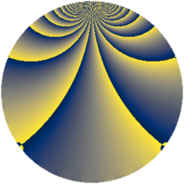# Properties

 Label 2240.2.cvLevel $2240$ Weight $2$ Character orbit 2240.cv Rep. character $\chi_{2240}(207,\cdot)$ Character field $\Q(\zeta_{12})$ Dimension $368$ Sturm bound $768$

# Related objects

## Defining parameters

 Level: $$N$$ $$=$$ $$2240 = 2^{6} \cdot 5 \cdot 7$$ Weight: $$k$$ $$=$$ $$2$$ Character orbit: $$[\chi]$$ $$=$$ 2240.cv (of order $$12$$ and degree $$4$$) Character conductor: $$\operatorname{cond}(\chi)$$ $$=$$ $$560$$ Character field: $$\Q(\zeta_{12})$$ Sturm bound: $$768$$

## Dimensions

The following table gives the dimensions of various subspaces of $$M_{2}(2240, [\chi])$$.

Total New Old
Modular forms 1600 400 1200
Cusp forms 1472 368 1104
Eisenstein series 128 32 96

## Trace form

 $$368q + 4q^{3} - 2q^{5} + 8q^{7} - 168q^{9} + O(q^{10})$$ $$368q + 4q^{3} - 2q^{5} + 8q^{7} - 168q^{9} + 4q^{11} - 24q^{15} - 4q^{17} - 8q^{21} + 4q^{23} - 8q^{27} - 4q^{33} + 26q^{35} + 12q^{45} - 24q^{47} + 4q^{51} - 4q^{53} + 16q^{55} + 24q^{57} - 16q^{59} - 4q^{61} - 12q^{63} - 4q^{65} + 24q^{69} + 96q^{71} - 10q^{75} + 20q^{77} - 128q^{81} + 16q^{83} - 28q^{85} + 4q^{87} + 24q^{91} - 16q^{97} - 24q^{99} + O(q^{100})$$

## Decomposition of $$S_{2}^{\mathrm{new}}(2240, [\chi])$$ into newform subspaces

The newforms in this space have not yet been added to the LMFDB.

## Decomposition of $$S_{2}^{\mathrm{old}}(2240, [\chi])$$ into lower level spaces

$$S_{2}^{\mathrm{old}}(2240, [\chi]) \cong$$ $$S_{2}^{\mathrm{new}}(560, [\chi])$$$$^{\oplus 3}$$